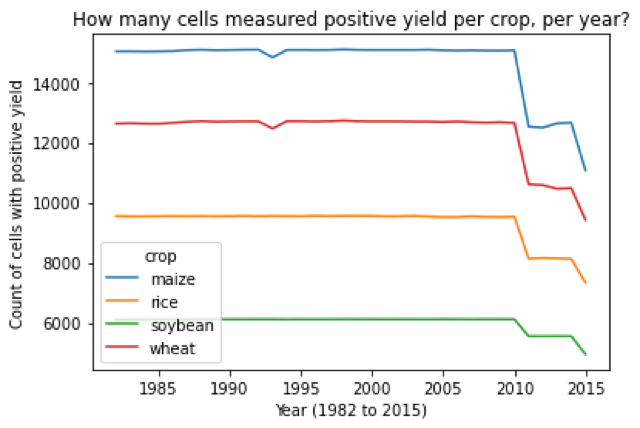# JUNTO Practice: Data Analysis, Historical Crop Yields (Part 3)

Discussed on December 01, 2020.

Analyze this dataset and create a short report:

Click to see:

# How many measurements are taken, per year, per crop?

I look at crop data from 1982 to 2015 for maize, rice, soybean, and wheat. I count the number of “cells” with positive yield, and I find that there are significant decreases in 2010-2011 and 2014-2015. The decrease in 2010-2011 is explained by a change in dataset (version 1.2 to version 1.3), but the decrease in 2014-2015 is unexplained. Future research should use geospatial visualization on these years to understand how the decrease manifests.

``````import json

with open("config.json") as file:
``````

This config file has an entry for the “data directory” that holds the historical crop yield data.

## Load historical crop yield data and count cells with positive yield

I load crop data from 1982 to 2015 for 4 major crops: maize, rice, soybean, and wheat. I ignore the data from 1986 and 2016 because Izumi (2019) explains that there is significant missing data in these years.

``````from itertools import product
from pathlib import Path

import netCDF4
import numpy as np
import pandas as pd

data_dir = Path(CONFIG["data_dir"])

domain_crop = "maize", "rice", "soybean", "wheat"
domain_year = range(1982, 2016)

rows = []

for crop, year in product(domain_crop, domain_year):
data_path = data_dir / crop / f"yield_{year}.nc4"
assert data_path.is_file()
with netCDF4.Dataset(data_path) as data:
yield_ = np.array(data["var"])
n_yield = (yield_ > 0).sum()
rows.append((crop, year, n_yield))

df = pd.DataFrame(rows, columns=["crop", "year", "n_yield"])
``````

Here’s a sample of this data:

``````df.sample(10)
``````
``````        crop  year  n_yield
81   soybean  1995     6120
128    wheat  2008    12660
56      rice  2004     9538
16     maize  1998    15099
90   soybean  2004     6119
102    wheat  1982    12628
87   soybean  2001     6120
125    wheat  2005    12683
13     maize  1995    15081
55      rice  2003     9558
``````

## Graphing the count of cells with positive yield

Here’s a plot of the “count of cells with positive yield” per crop, per year:

``````df.pivot(index="year", columns="crop").droplevel(
0, axis=1
).plot(
title="How many cells measured positive yield per crop, per year?",
xlabel=f"Year ({df['year'].min()} to {df['year'].max()})",
ylabel="Count of cells with positive yield",
)
``````
``````<matplotlib.axes._subplots.AxesSubplot at 0x7faca0e00190>
``````From this graph, I can see that the “count of cells with positive yield” is relatively constant from 1982 to 2010. But there is a large decrease in both 2010-2011 and 2014-2015.

• For 2010-2011, Izumi (2019) switches from using Version 1.2 to Version 1.3 of the “Global Dataset of Historical Yield”. I think that this explains the decrease in cell count for 2010-2011.
• But for 2014-2015, I’m not sure what explains the decrease in cell count.

Further research should do a geospatial visualization of the data for both 2010-2011 and 2014-2015. I believe that this visual will help me understand how the data changes so drastically.